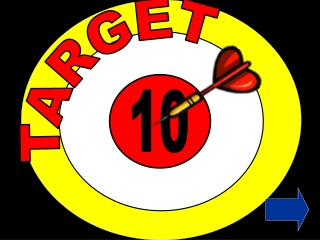Download PresentationTARGET

# TARGET

Download Presentation## TARGET

- - - - - - - - - - - - - - - - - - - - - - - - - - - E N D - - - - - - - - - - - - - - - - - - - - - - - - - - -
##### Presentation Transcript

1. TARGET 10

2. 0 9 1 8 2 10 7 3 6 4 5 NO YES

3. 8 + 2 CHECK! Count the arrows to add up the numbers +2 8 9 10 0 1 2 3 4 5 6 7 8+ 2 = 10 2+ 8 = 10

4. 0 9 1 8 2 10 7 3 6 4 5 NO YES

5. 4 + 3 CHECK! Count the arrows to add up the numbers +3 6 7 4 5 0 1 2 3 8 9 10 4+ 3 = 7

6. 0 9 1 8 2 10 7 3 6 4 5 NO YES

7. 5 + 5 CHECK! Count the arrows to add up the numbers +5 5 6 7 8 9 10 0 1 2 3 4 5+ 5 = 10

8. 0 9 1 8 2 10 7 3 6 4 5 NO YES

9. 6 + 4 CHECK! Count the arrows to add up the numbers +4 6 7 8 9 10 0 1 2 3 4 5 6+ 4 = 10 4+ 6 = 10

10. 0 9 1 8 2 10 7 3 6 4 5 NO YES

11. 7 + 2 CHECK! Count the arrows to add up the numbers +2 7 8 9 10 0 1 2 3 4 5 6 7+ 2 = 9

12. 0 9 1 8 2 10 7 3 6 4 5 NO YES

13. 10 + 0 CHECK! Count the arrows to add up the numbers When adding 0 the number does not change 10 0 1 2 3 4 5 6 7 8 9 10+ 0 = 10 0+ 10 = 10

14. 0 9 1 8 2 10 7 3 6 4 5 NO YES

15. 6 + 2 CHECK! Count the arrows to add up the numbers +2 6 7 8 0 1 2 3 4 5 9 10 6+ 2 = 8

16. 0 9 1 8 2 10 7 3 6 4 5 NO YES

17. 7 + 3 CHECK! Count the arrows to add up the numbers +3 7 8 9 10 0 1 2 3 4 5 6 7+ 3 = 10 3+ 7 = 10

18. 0 9 1 8 2 10 7 3 6 4 5 NO YES

19. 9 + 1 CHECK! Count the arrows to add up the numbers +1 9 10 0 1 2 3 4 5 6 7 8 1+ 9 = 10 9+ 1 = 10

20. NUMBER BONDS OF 10 10 + 0 = 10 0 + 10 = 10 9 + 1 = 10 1 + 9 = 10 8 + 2 = 10 2 + 8 = 10 7 + 3 = 10 3 + 7 = 10 6 + 4 = 10 4 + 6 = 10 5 + 5 = 10 WRITE THESE DOWN

21. WELL DONE 10 I AM A NUMBER BOND EXPERT